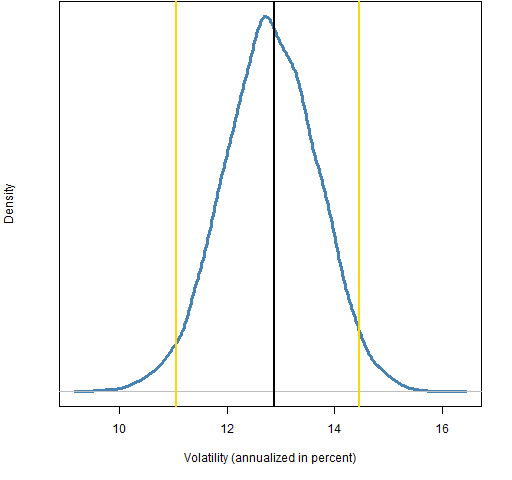Want to share your content on R-bloggers? click here if you have a blog, or here if you don't.

Investment Performance Guy has a post “Periodicity of risk statistcs (and other measures)” in which it is wondered how valid volatility estimates are from a month of daily returns.

Here is a quick look.  Figure 1 shows the variability (and a 95% confidence interval) of volatility estimates for the S&P 500 index in January 2011.  Figure 2 is for the first quarter and Figure 3 is for the first half.  All of these are with daily data.

Figure 1: Volatility and bootstrap distribution for January 2011 volatility of the S&P 500.Figure 2: Volatility and bootstrap distribution for Q1 of 2011 volatility of the S&P 500.Figure 3: Volatility and bootstrap distribution for H1 of 2011 volatility of the S&P 500.## Appendix R

The bootstrapping is done like:

for(i in 1:1e4) spxvolQ1.boot[i] <- sd(spxret11Q1[sample(62,62, replace=TRUE)])

The plots are something like:

plot(density(spxvolM1.boot)*100*sqrt(252))

Subscribe to the Portfolio Probe blog by Email